# Basic Logic Gates

## Digital Circuits

• #### Question 1

Identify the type of logic gate shown in this schematic diagram, and explain why it has the name it does:• #### Question 2

Identify the type of logic gate shown in this schematic diagram, and explain why it has the name it does:• #### Question 3

Identify the type of logic gate shown in this schematic diagram, and explain why it has the name it does: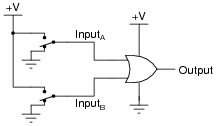• #### Question 4

Crude logic gates circuits may be constructed out of nothing but diodes and resistors. Take for example this logic gate circuit: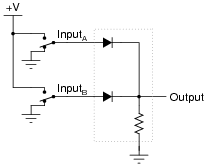Identify what type of logic function is represented by this gate circuit (AND, OR, inverter, etc.).

• #### Question 5

Crude logic gates circuits may be constructed out of nothing but diodes and resistors. Take for example this logic gate circuit: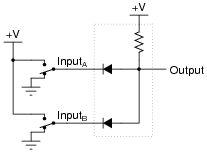Identify what type of logic function is represented by this gate circuit (AND, OR, inverter, etc.). Also, trace the directions of all currents in this circuit.

• #### Question 6

Identify each of these logic gates by name, and complete their respective truth tables:• #### Question 7

One way to think of the basic logic gate types (all but the XOR and XNOR gates) is to consider what single input state guarantees a certain output state. For example, we could describe the function of an OR gate as such:

 Any high input guarantees a high output.

Identify what type of gate is represented by each of the following phrases:

Any high input guarantees a low output.
Any low input guarantees a high output.
Any low input guarantees a low output.
• #### Question 8

One way to think of logic gate types is to consider what input states guarantee a certain output state. For example, we could describe the function of an AND gate as such:

 Any low input guarantees a low output.

Identify what type of gate is represented by each of the following phrases:

Any low input guarantees a high output.
Any high input guarantees a low output.
Any high input guarantees a high output.
Any difference in the inputs guarantees a high output.
Any difference in the inputs guarantees a low output.

Also, explain how this sort of gate identification could be useful in troubleshooting logic gate circuits.

• #### Question 9

A different way to view the functions of two-input logic gates is to think of them in terms of signal controllers, where the status of one input affects how the other input’s signal passes through to the output. The generic schematic diagram for this format is as such: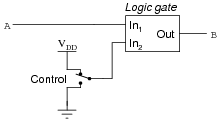Identify the types of logic gates which do the following (there is more than one type of gate for each of the following rules!):

B = A when Control is high
B = A when Control is low
B = $$\bar{A}$$ when Control is high
B = $$\bar{A}$$ when Control is low

Also, explain how an understanding of this can be helpful in troubleshooting faulted logic gates.

• #### Question 10

Many types of logic gate circuits are built with more than two inputs. These are useful, even necessary, in some digital circuit applications. Research the part numbers and datasheets of the following logic gate integrated circuits:

Triple 3-input NOR gate
Dual 4-input AND gate
Single 8-input NAND gate
• #### Question 11

Complete the truth table for a three-input AND gate: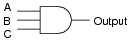A B C Output 0 0 0 0 0 1 0 1 0 0 1 1 1 0 0 1 0 1 1 1 0 1 1 1

• #### Question 12

Suppose you needed a two-input AND gate, but happened to have an unused 3-input AND gate in one of the integrated circuits (“chips”) already in the system you were building. Of course, you could just add another IC containing 2-input AND gates, but it seems a shame to waste the 3-input gate already there.

Explain what you would need to do with the third input terminal on this gate in order to use it as a 2-input AND gate: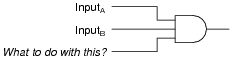Now, explain what to do with each of the following gates’ third inputs, in order to use each of them as 2-input gates: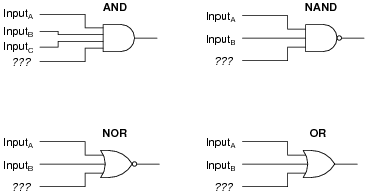In each case, describe why your solution works.